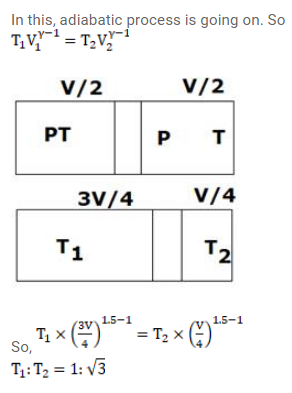# Fig. shows a cylindrical tube with adiabatic walls and fitted withQuestion:

Fig. shows a cylindrical tube with adiabatic walls and fitted with an adiabatic separator. The separator can be slid into the tube by an external mechanism. An ideal gas $(\gamma=1.5)$ is injected in the two sides at equal pressures and temperatures. The separator remains in equilibrium at the middle. It is now slid to a position where it divides the tube in the ratio $1: 3$. Find the ratio of the temperatures in the two parts of the vessel.Solution: